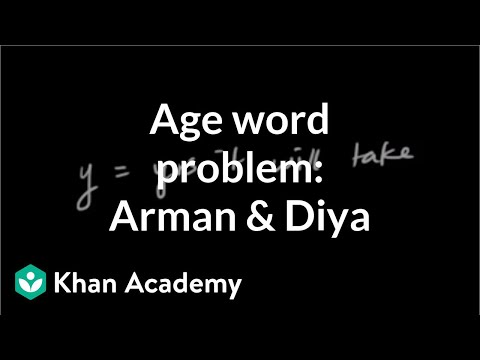Video

# Completing solutions to 2-variable equations (Full video)

Description: Given a 2-variable equation and the x or y values of a solution, Sal finds the value of the other variable in the solution. And they give us the equation, and then they want us to figure out, what does y equal when x is equal to negative five? And to figure this out, I've actually copied and pasted this part of the problem onto my scratchpad, so let me get that out. Actually, let me do it the second way, first.

### Other videos you might be interested in### Solving Systems of Equations by Substitution (Full video)

#### Student Achievement Partners